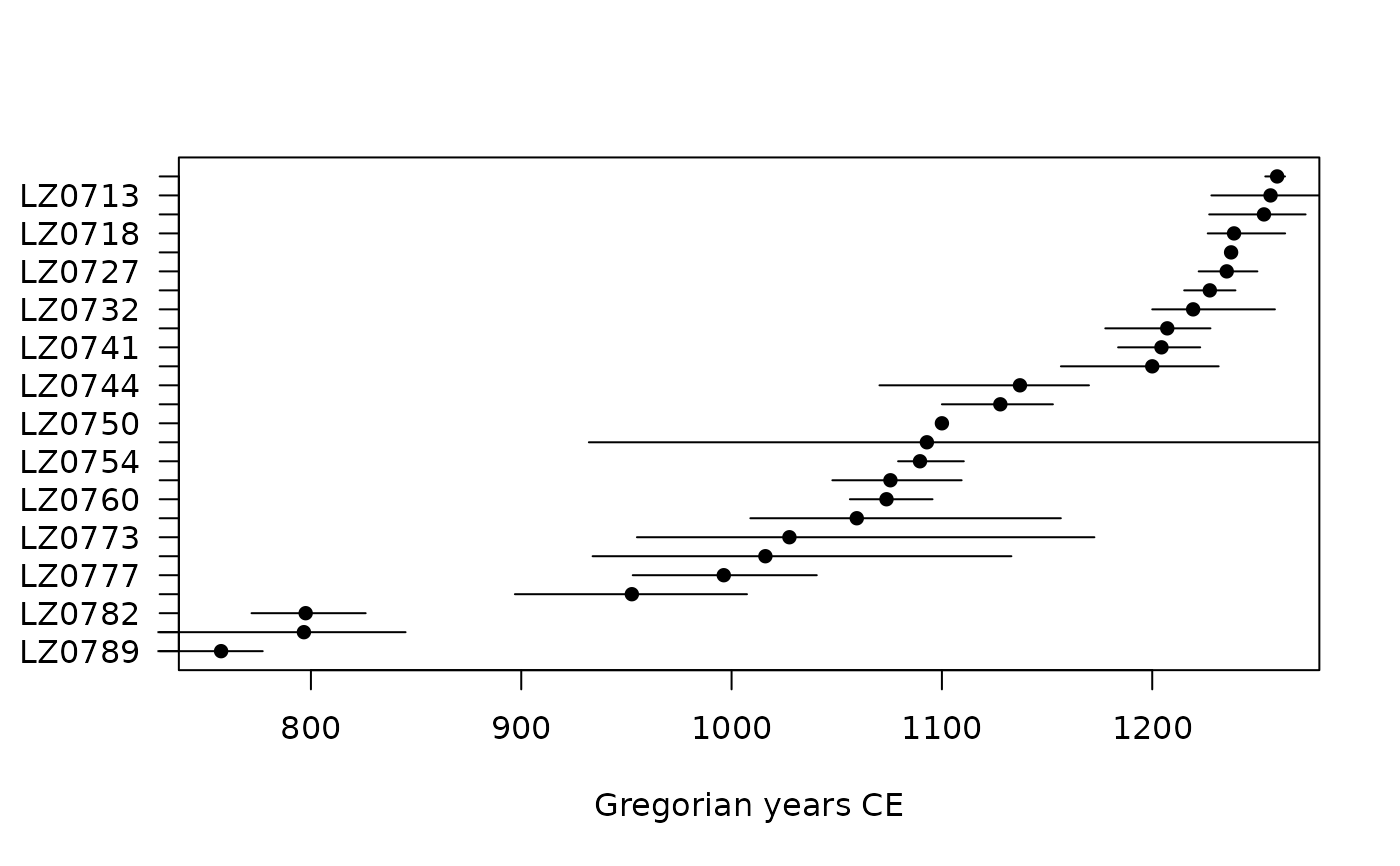Estimates the Mean Ceramic Date of an assemblage.

Usage

mcd(object, dates, ...)

# S4 method for numeric,numeric
mcd(object, dates, na.rm = FALSE)

# S4 method for CountMatrix,numeric
mcd(object, dates)

# S4 method for MeanDate
bootstrap(
object,
level = 0.95,
type = c("student", "normal"),
probs = c(0.25, 0.5, 0.75),
n = 1000
)

# S4 method for MeanDate
jackknife(object)

Arguments

object

A numeric vector, a arkhe::CountMatrix or a MeanDate object.

dates

A numeric vector of dates.

...

Currently not used.

na.rm

A logical scalar: should missing values (including NaN) be removed?

level

A length-one numeric vector giving the confidence level. Must be a single number between $$0$$ and $$1$$. If NULL, no confidence interval are computed.

type

A character string giving the type of confidence interval to be returned. It must be one "student" (default) or "normal". Any unambiguous substring can be given. Only used if level is not NULL.

probs

A numeric vector of probabilities with values in $$[0,1]$$ (see stats::quantile()). If NULL, quantiles are not computed.

n

A non-negative integer giving the number of bootstrap replications.

Details

The Mean Ceramic Date (MCD) is a point estimate of the occupation of an archaeological site (South 1977). The MCD is estimated as the weighted mean of the date midpoints of the ceramic types (based on absolute dates or the known production interval) found in a given assemblage. The weights are the relative frequencies of the respective types in the assemblage.

A bootstrapping procedure is used to estimate the confidence interval of a given MCD. For each assemblage, a large number of new bootstrap replicates is created, with the same sample size, by resampling the original assemblage with replacement. MCDs are calculated for each replicates and upper and lower boundaries of the confidence interval associated with each MCD are then returned.

South, S. A. (1977). Method and Theory in Historical Archaeology. New York: Academic Press.

Examples

## Mean Ceramic Date
## Coerce the zuni dataset to an abundance (count) matrix
data("zuni", package = "folio")
counts <- as_count(zuni)

## Set the start and end dates for each ceramic type
dates <- list(
LINO = c(600, 875), KIAT = c(850, 950), RED = c(900, 1050),
GALL = c(1025, 1125), ESC = c(1050, 1150), PUBW = c(1050, 1150),
RES = c(1000, 1200), TULA = c(1175, 1300), PINE = c(1275, 1350),
PUBR = c(1000, 1200), WING = c(1100, 1200), WIPO = c(1125, 1225),
SJ = c(1200, 1300), LSJ = c(1250, 1300), SPR = c(1250, 1300),
PINER = c(1275, 1325), HESH = c(1275, 1450), KWAK = c(1275, 1450)
)

## Calculate date midpoints
mid <- vapply(X = dates, FUN = mean, FUN.VALUE = numeric(1))

## Calculate MCD
mc_dates <- mcd(counts, dates = mid)
#> LZ1105 LZ1103 LZ1100 LZ1099 LZ1097 LZ1096
#>   1162   1138   1154   1091   1092    841

## Plot
plot(mc_dates, select = 100:125)## Bootstrap resampling
boot <- bootstrap(mc_dates, n = 30)
#>         min      mean  max     lower     upper     Q25    Q50     Q75
#> LZ1105 1119 1163.5667 1211 1156.4192 1170.7142 1152.25 1166.5 1173.00
#> LZ1103 1070 1134.5333 1189 1125.0235 1144.0431 1116.25 1131.5 1153.75
#> LZ1100 1075 1156.2667 1195 1144.5596 1167.9738 1140.00 1171.5 1179.00
#> LZ1099 1081 1091.5333 1100 1089.7446 1093.3221 1088.75 1091.0 1094.00
#> LZ1097 1028 1079.0667 1156 1064.3529 1093.7804 1055.00 1073.0 1111.00
#> LZ1096  738  834.1667  945  812.7585  855.5748  789.00  841.0  893.00

## Jackknife resampling
jack <- jackknife(mc_dates)